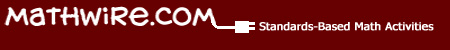Template Library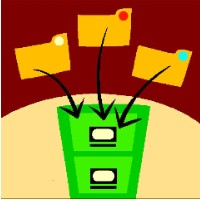## Template Library Categories

• to increase student participation in math lessons
• to assess student proficiency and mathematical understanding of concepts and skills## Number & Operations Templates

•Hundred Board Template for students to use for skip-counting exercises, problem solving and addition or subtraction number stories.
•Small Hundred Grids Template for student desks or for use in solving hundred board logic problems
•Small Student Numbers 1-100 for student use in number sense activities and to build the hundred board
•Ten-Frame Template for students to use with counters or to record number stories using dry-erase markers.
•Double Ten-Frame Template for representing numbers 1-20.
•Domino Template for students to use with counters or to create number stories using dry-erase markers.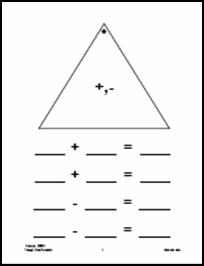### Facts Practice & Number Stories

•Domino Facts Template for students to use with counters or to create number stories using dry-erase markers.
•Fact Family Template for students to use to practice writing fact families.
•Triangle Cards Template for students to use to write fact families as they are found on the triangle cards that are commercially available.
•Blank Wheel Template for students to addition, subtraction, multiplication or division facts.
•Ladybug Template for students to use with counters or to create number stories using dry-erase markers.
•Climb the Ladder Template for students to use to write equivalent names for specified numbers.

### Place Value Templates

•Tens-Ones Mat Template for students to use with base-ten or groupable materials.
•Tens-Ones Recording Template for students to record and represent concrete work with base-ten manipulatives.
•Place Value Template for students to record dictated numbers.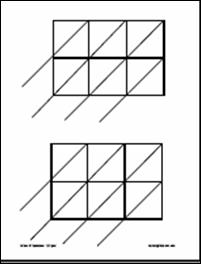### Fraction, Decimal & Percent Templates

•Fraction Circles Template: circle area model so that students can draw fractions.
•Fraction Squares Template: square area model so that students can draw fractions.
•Fraction Rectangles Template: rectangle area model so that students can draw fractions.
•Fraction Circles Template: circle area model so that students can draw fractions.
•Decimal Squares Template: 10x10 grid model so that students can shade in decimals and relate the decimal to fraction and percent.
•Decimal Place Value: to thousandths place for students to practice writing dictated decimals.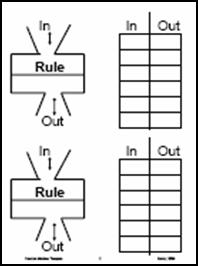## Patterns & Algebra Templates

•Magic Pot Workmat for students to use in sheet protectors as a follow-up to reading Two of Everything, a humorous introduction to functions and function machines.   This mat is also used to record input and output numbers in the Guess My Rule game.
•Magic Pot Mat: use with linking cubes of two colors to reinforce in/out and rule.
•Function Machine Template can be placed in a sheet protector so that students can use dry-erase markers to play the game by recording input/output numbers, then erasing when they switch places.
•Generic 3-column Function Template
•Function: Table & Graph Template for student use in sleeve protectors to provide template for students to create a table of values and graph the values on the coordinate plane.
•Integer Mat Template: use with 2-color counters to concretely develop the positive-negative charge model of integers.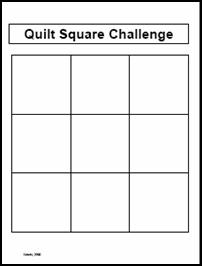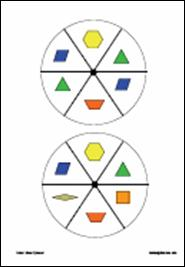## Data Analysis & Probability Templates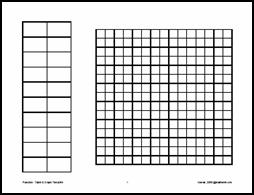•Bar Graph
•Blank Spinner/Bar Graph
•Assorted Spinners designed to be used with transparent spinner
•Pattern Block Spinners designed to be used with transparent spinner
•Frequency: Sum of Two Dice Template enables students to record the results of tossing a red and a blue die then tabulate the frequency of each sum.   This format helps students appreciate the fact that one can roll a 2 on the blue die and a 3 on the red die or a 3 on the blue die and a 2 on the die.   Although both will have a sum of 5, they represent two different outcomes.
•Frequency Table Template for students to use to create frequency tables in data collection.
•Frequency/Bar Graph Template for students to use to organize and graph data.
•Function-Graph Template enables students to record data and look for a functional relationship by plotting the points on the graph.
•Graph Paper Template: 1/2 inch

## Discrete Math Templates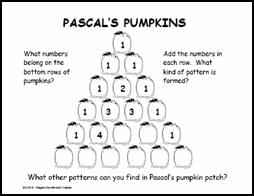•Pascal's Pumpkins   encourages students to look for patterns in Pascal's Triangle.   The handout develops awareness of this important mathematical pattern through this timely seasonal activity.   Students who take higher math courses will meet Pascal again in many different applications, including probability.   This handout is an outgrowth of the Rutgers Discrete Math Institute.
•Pascal's Penguins - 1 introduces primary students to a small version of Pascal's Triangle in this simple patterning activity.
•Pascal's Penguins - 2 introduces a larger version of Pascal's triangle and encourages students to identify the different patterns within the triangle and use these patterns to fill in the missing penguin numbers.
•Pascal's Hearts is a Valentine's Day application of Pascal's Triangle.